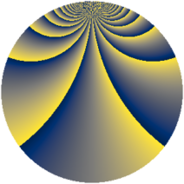# Properties

 Label 1815.2.btLevel $1815$ Weight $2$ Character orbit 1815.bt Rep. character $\chi_{1815}(7,\cdot)$ Character field $\Q(\zeta_{220})$ Dimension $10560$ Sturm bound $528$

# Related objects

## Defining parameters

 Level: $$N$$ $$=$$ $$1815 = 3 \cdot 5 \cdot 11^{2}$$ Weight: $$k$$ $$=$$ $$2$$ Character orbit: $$[\chi]$$ $$=$$ 1815.bt (of order $$220$$ and degree $$80$$) Character conductor: $$\operatorname{cond}(\chi)$$ $$=$$ $$605$$ Character field: $$\Q(\zeta_{220})$$ Sturm bound: $$528$$

## Dimensions

The following table gives the dimensions of various subspaces of $$M_{2}(1815, [\chi])$$.

Total New Old
Modular forms 21440 10560 10880
Cusp forms 20800 10560 10240
Eisenstein series 640 0 640

## Trace form

 $$10560q + 8q^{5} + 20q^{7} + O(q^{10})$$ $$10560q + 8q^{5} + 20q^{7} - 44q^{10} - 8q^{11} + 16q^{12} - 44q^{13} + 12q^{15} - 264q^{16} + 20q^{17} + 60q^{20} - 56q^{22} - 32q^{23} + 32q^{25} - 16q^{26} + 60q^{28} + 40q^{30} - 16q^{31} + 16q^{33} - 264q^{36} + 124q^{37} - 56q^{38} + 120q^{41} - 12q^{42} + 200q^{46} - 60q^{47} - 48q^{48} + 140q^{50} - 40q^{51} - 40q^{52} - 36q^{53} + 96q^{55} - 384q^{56} - 40q^{57} - 68q^{58} - 48q^{60} - 40q^{61} - 80q^{62} - 20q^{63} - 56q^{66} - 192q^{67} - 80q^{68} - 12q^{70} + 60q^{73} - 16q^{75} + 264q^{76} + 24q^{77} + 120q^{80} + 2640q^{81} - 8q^{82} + 200q^{83} - 96q^{85} + 64q^{86} + 144q^{88} - 24q^{91} - 420q^{92} + 72q^{93} - 60q^{95} - 32q^{97} + O(q^{100})$$

## Decomposition of $$S_{2}^{\mathrm{new}}(1815, [\chi])$$ into newform subspaces

The newforms in this space have not yet been added to the LMFDB.

## Decomposition of $$S_{2}^{\mathrm{old}}(1815, [\chi])$$ into lower level spaces

$$S_{2}^{\mathrm{old}}(1815, [\chi]) \cong$$ $$S_{2}^{\mathrm{new}}(605, [\chi])$$$$^{\oplus 2}$$